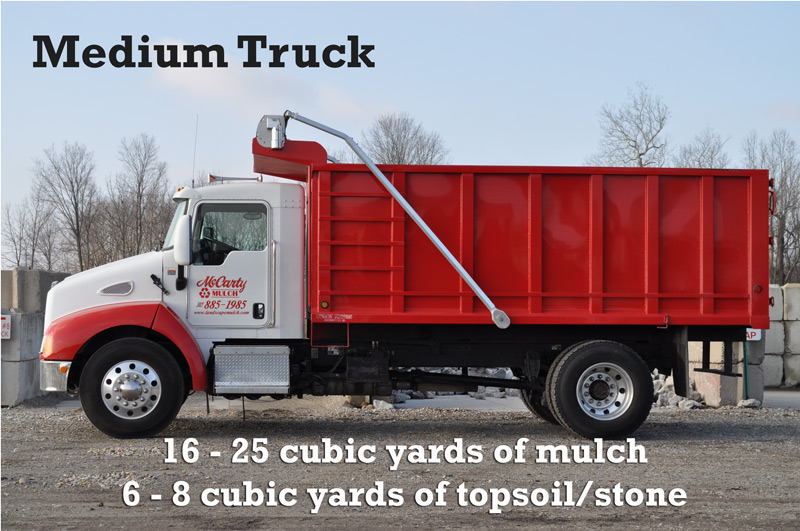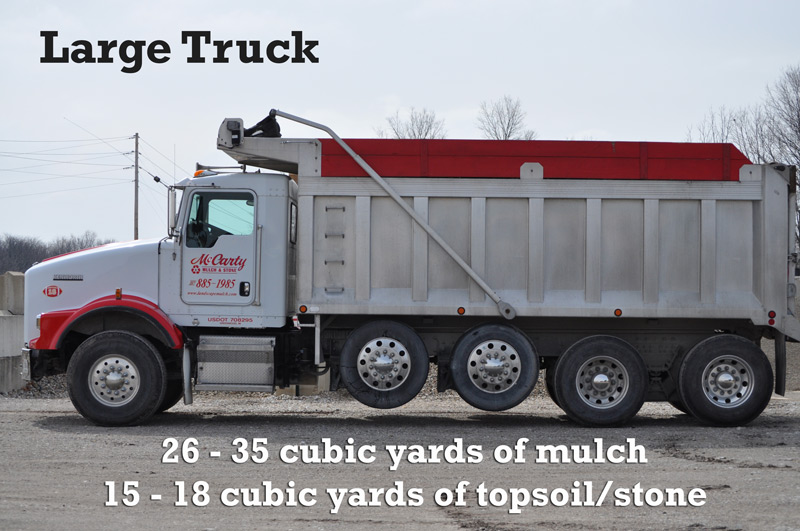# Mulch Calculator

(that includes a right or 90° angle)
Measure the length of the two sides that meet at the right angle

## You will need approximately: X cubic yards.

### MULCH CALCULATOR/YARDAGE

McCarty Mulch & Stone loads and sells all of our bulk products by the cubic yard. A cubic yard is a volume measurement with dimensions of 3 ft. x 3 ft. x 3 ft. There are 27 cubic feet in a cubic yard.

To get a good estimate of the yardage amount you need, you must first come up with square footage.

Basically length of the area X the width of the area. Length X Width X Depth divided 27 = Cubic Yards

1″= .083
2″=.167
3″=.25
4″=.33
5″=.42
6″=.5

Estimated square feet coverage at a glance:
1 cubic yard will cover approximately 162 square feet at 2″ thickness.
1 cubic yard will cover approximately 108 square feet at 3″ thickness

##### Calculate number of stones

Looking for information on how to calculate the number of stones to use in your design.  Follow the instructions below to find the best way to get the right amount of stones to purchase.

1. Determine the face feet (width ft x length ft) of the area to cover.
2. Divide the area in sq. ft. by the number of square feet each stone covers to determine weight in tons. See individual stone descriptions.
3. Multiply the required number of tons by 2,000 lb to determine the total amount of pounds required.
4. Multiply the required number of pounds by the price per pound.

Note: Each pallet weights between 2,000-3,000 pounds depending on the stone type.

How to figure required amount if wall stone: Determine the face feet (width ft x length ft) of the area to cover.

1. Divide the area in sq. ft. by the number of square feet each stone covers to determine weight in tons.
2. Multiply the required number of tons by 2,000 lb to determine the total amount of pounds required.
3. Multiply the required number of pounds by the price per pound.

Note: Each pallet weights between 2,000-3,000 pounds depending on the stone type.

#### HOW TO USE THE CALCULATOR

Use our helpful mulch calculator to determine the amount of mulch you need for your landscape.

Its as easy as 1, 2, 3...

1. Select the thickness of your beds
2. Select Square/Rectangle, Circle or Triangle
3. Enter dimensions
4. Click Calculate

Looking for information on how to calculate the number of stones to use in your design.

#### Delivery Trucks